2022-03-0500

## 目录

First of all, install the PyCharm to configure my python environment.
Try to use the PyCharm to create my first Python program!
Let's code a REAL hello world! lolllll
Then try a input:
And some math?
Let's try some circle, btw we can use the:
let it print for one line.
If u want to input a num not a string as normal?
Do a math of a circle?
This is the point!
How to print a num in Binary/Octal/Hex!
Here it is!
Let's do some big!
Try to find the MAX/MIN/AVERAGE num from a num list!
ALL IS GOOD!
And this is my first lesson to study Python, I got really much, see u next time!The core of extensible programming is defining functions. Python allows mandatory and optional arguments, keyword arguments, and even arbitrary argument lists.###### Try to use the PyCharm to create my first Python program!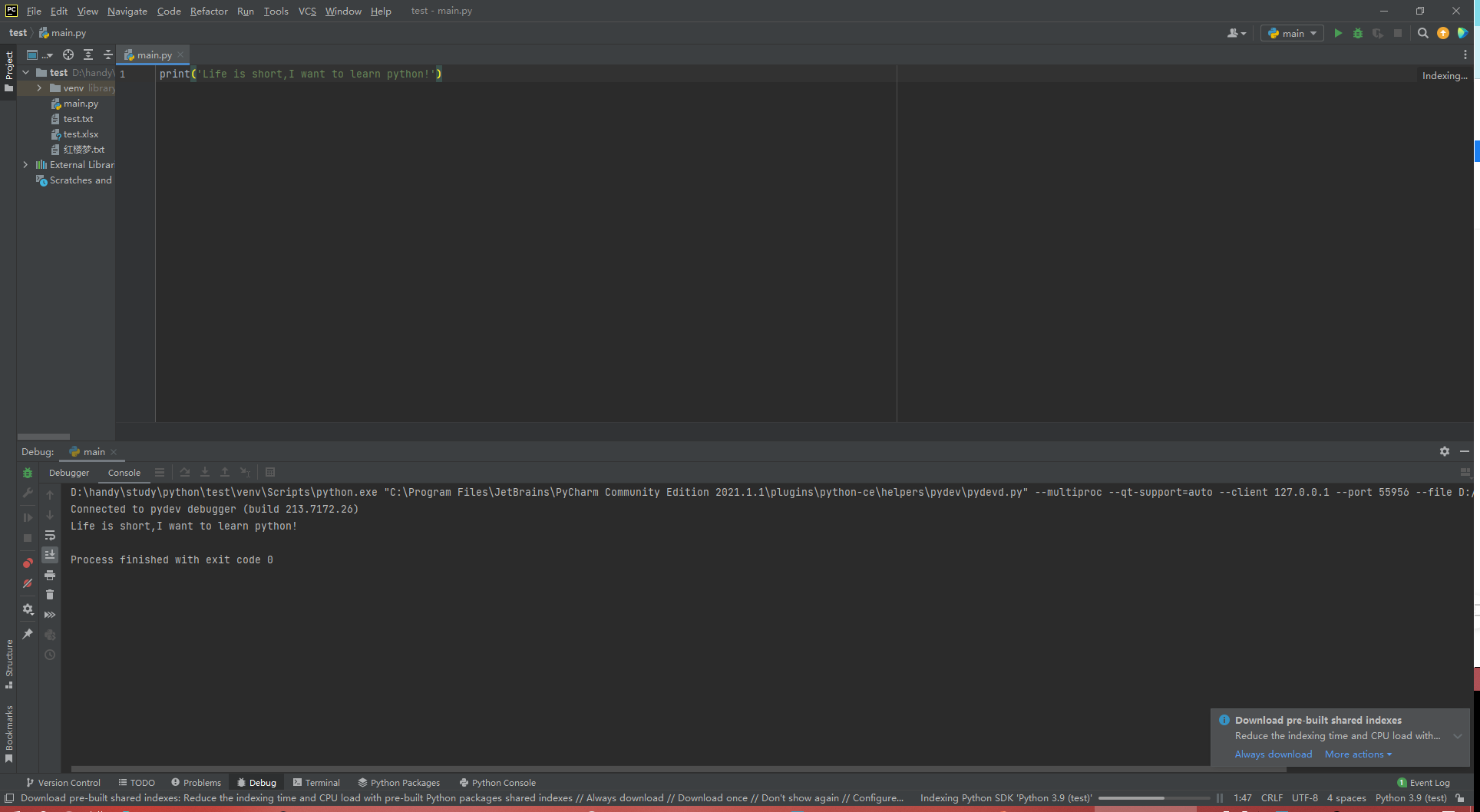(That is not only the Hello world program, but also the big step to study the Python!XD

###### Let's code a REAL hello world! lolllll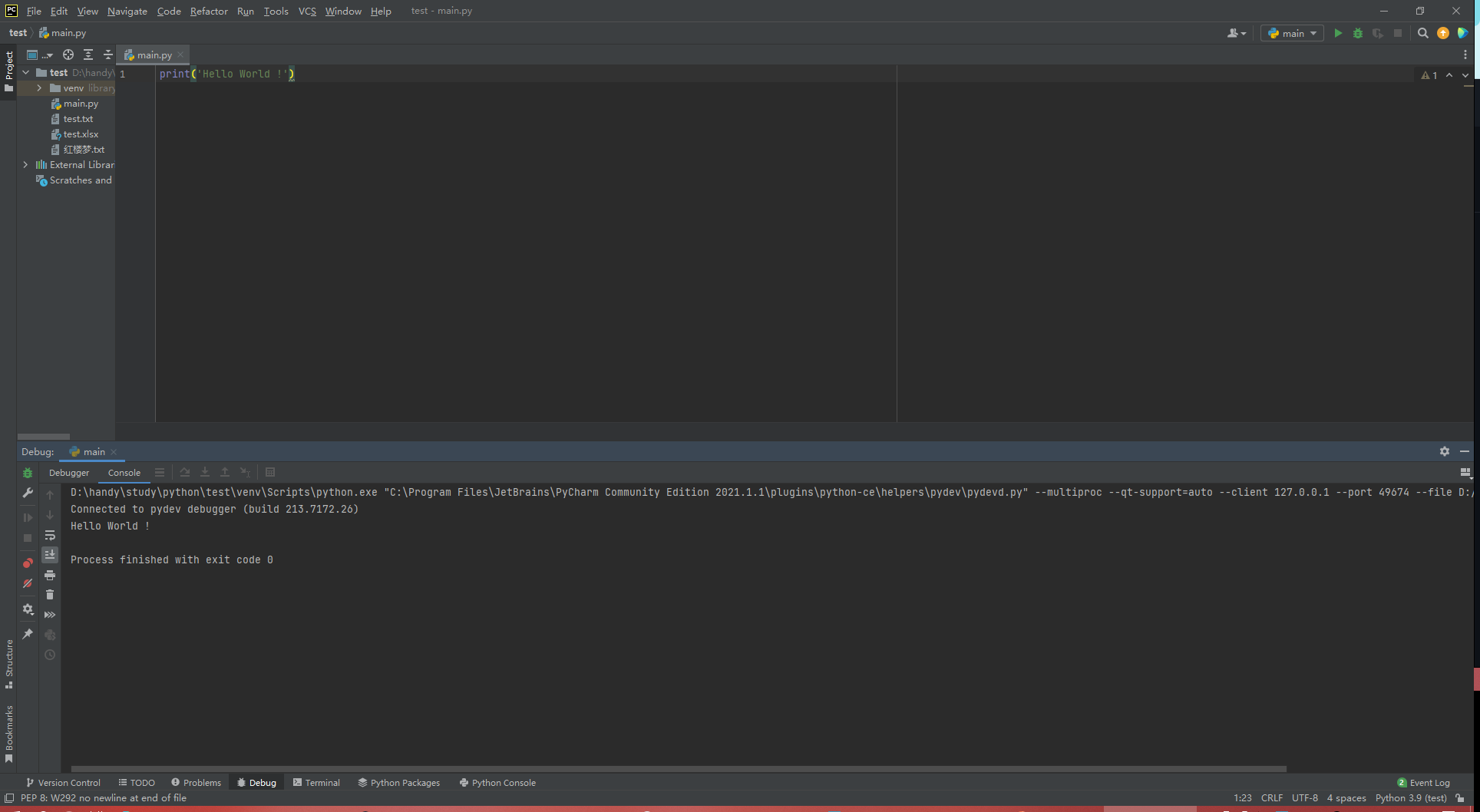(U can use the SHIFT + F9 to encode the codes by keyboard)

###### Then try a input: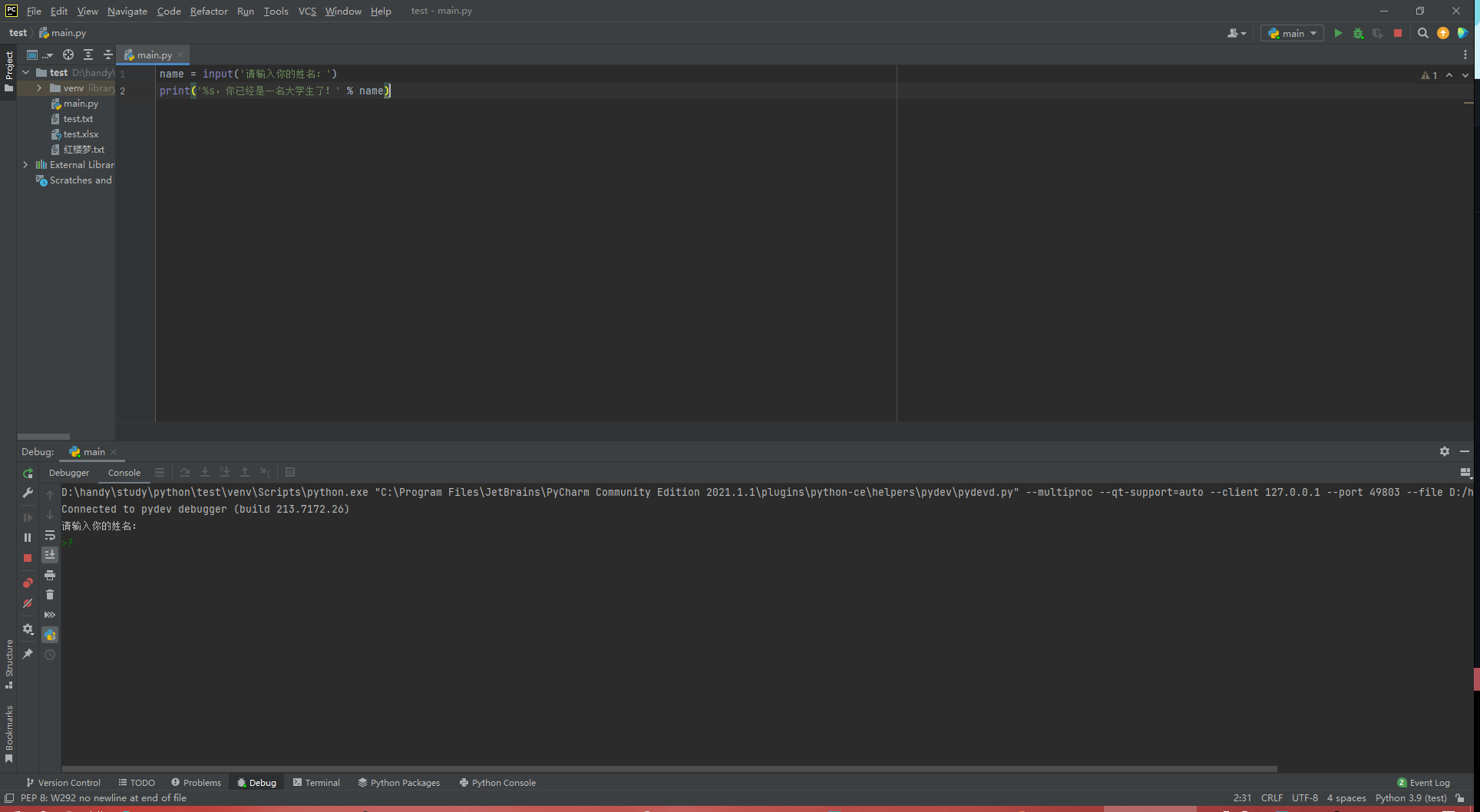###### And some math?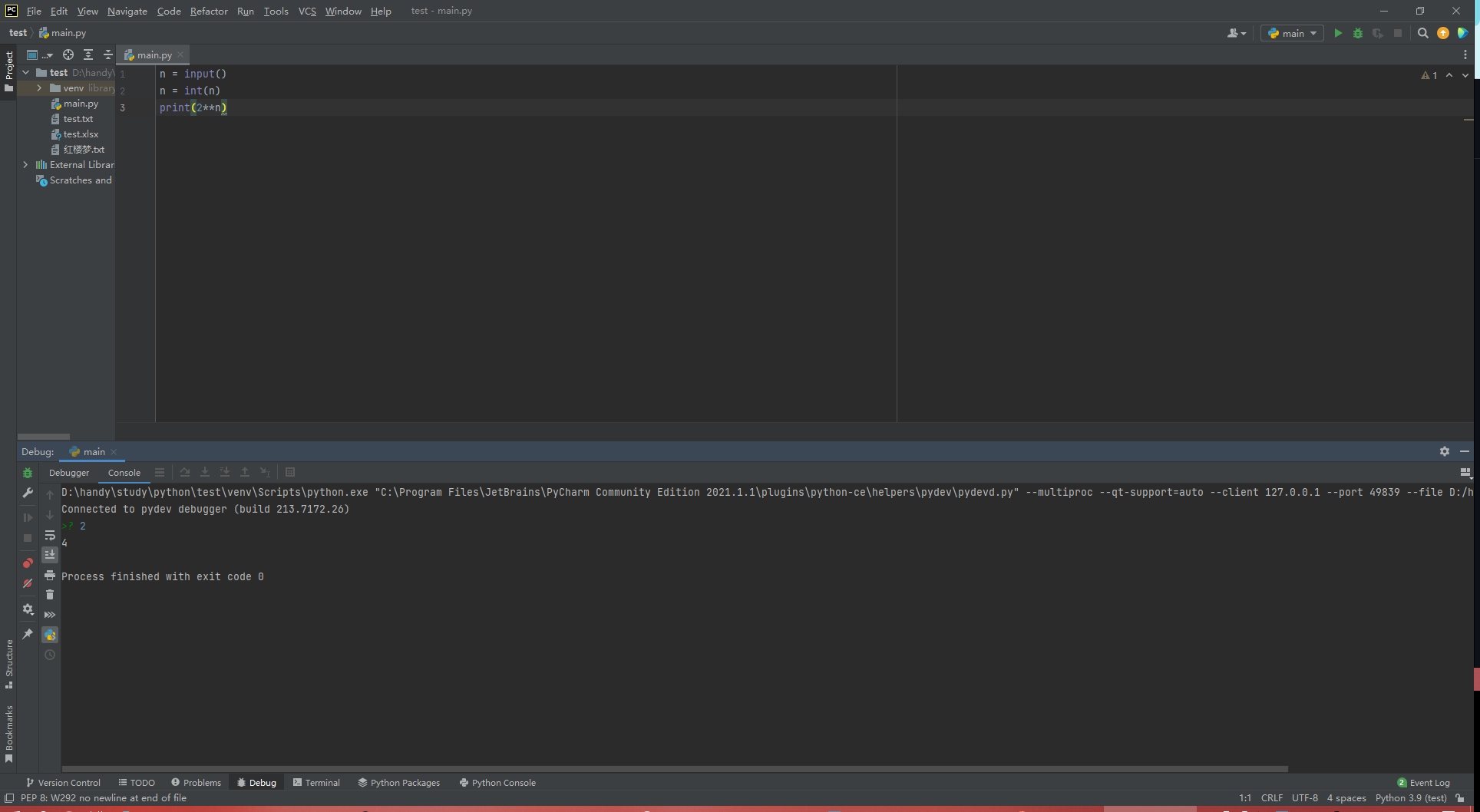###### Let's try some circle, btw we can use the:

`print(' ', end = ' ')`

###### let it print for one line.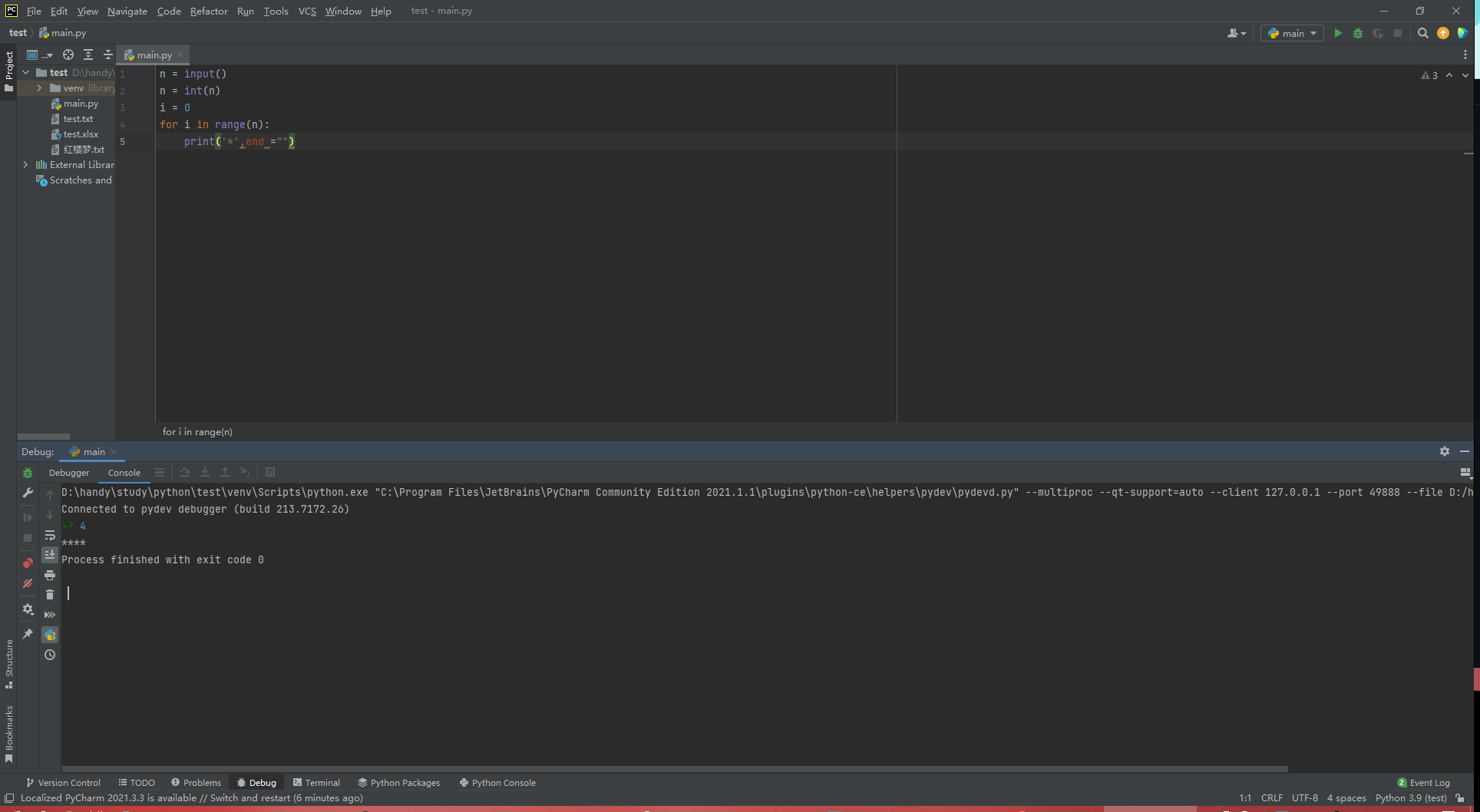###### If u want to input a num not a string as normal?

`r = int(input())`

###### Do a math of a circle?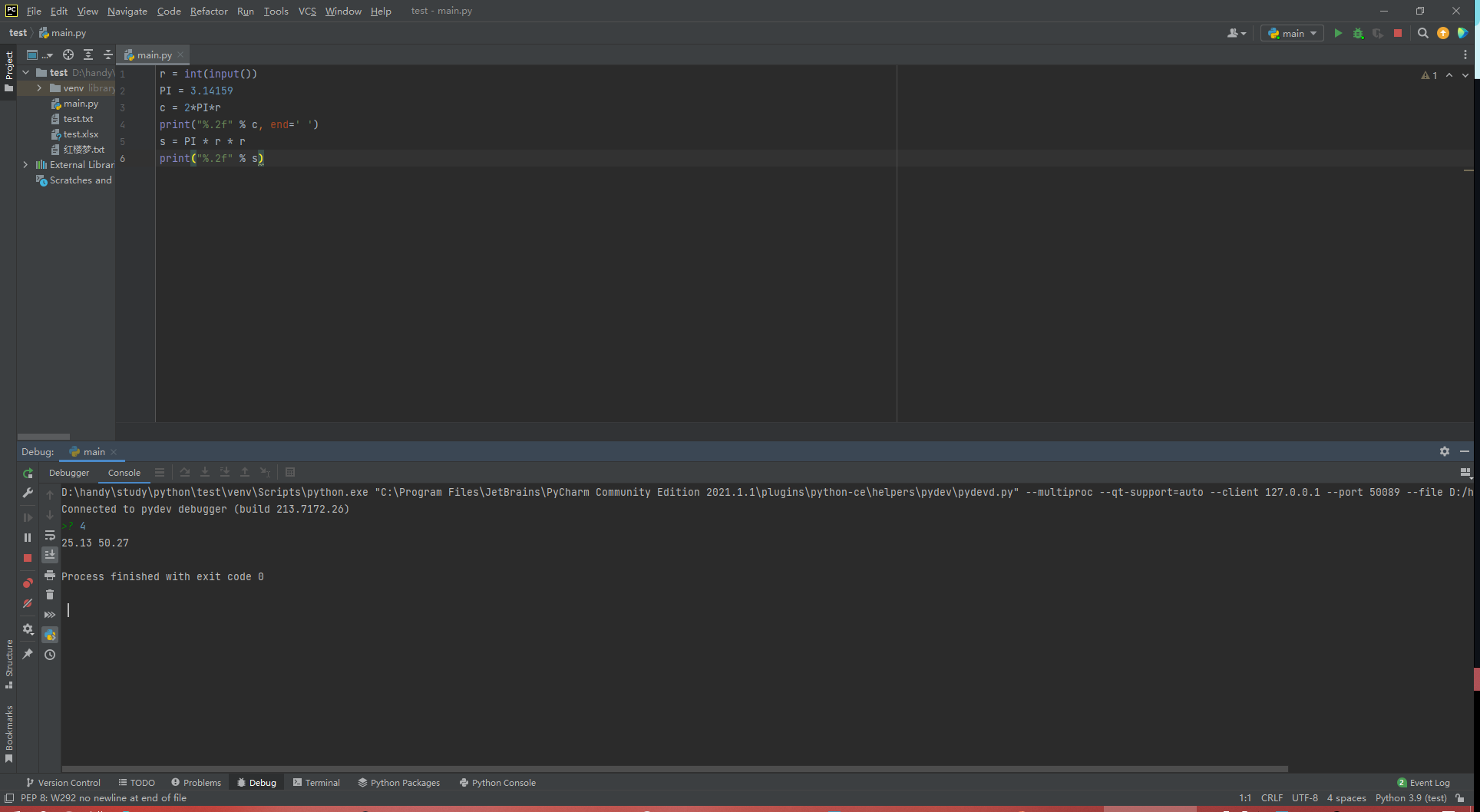##### This is the point!
###### How to print a num in Binary/Octal/Hex!

`a = int(input()) str1 = str(bin(a)) print(str1[2:], end=' ') str2 = str(oct(a)) print(str2[2:], end=' ') str3 = str(hex(a)) print(str3[2:])`

###### Here it is!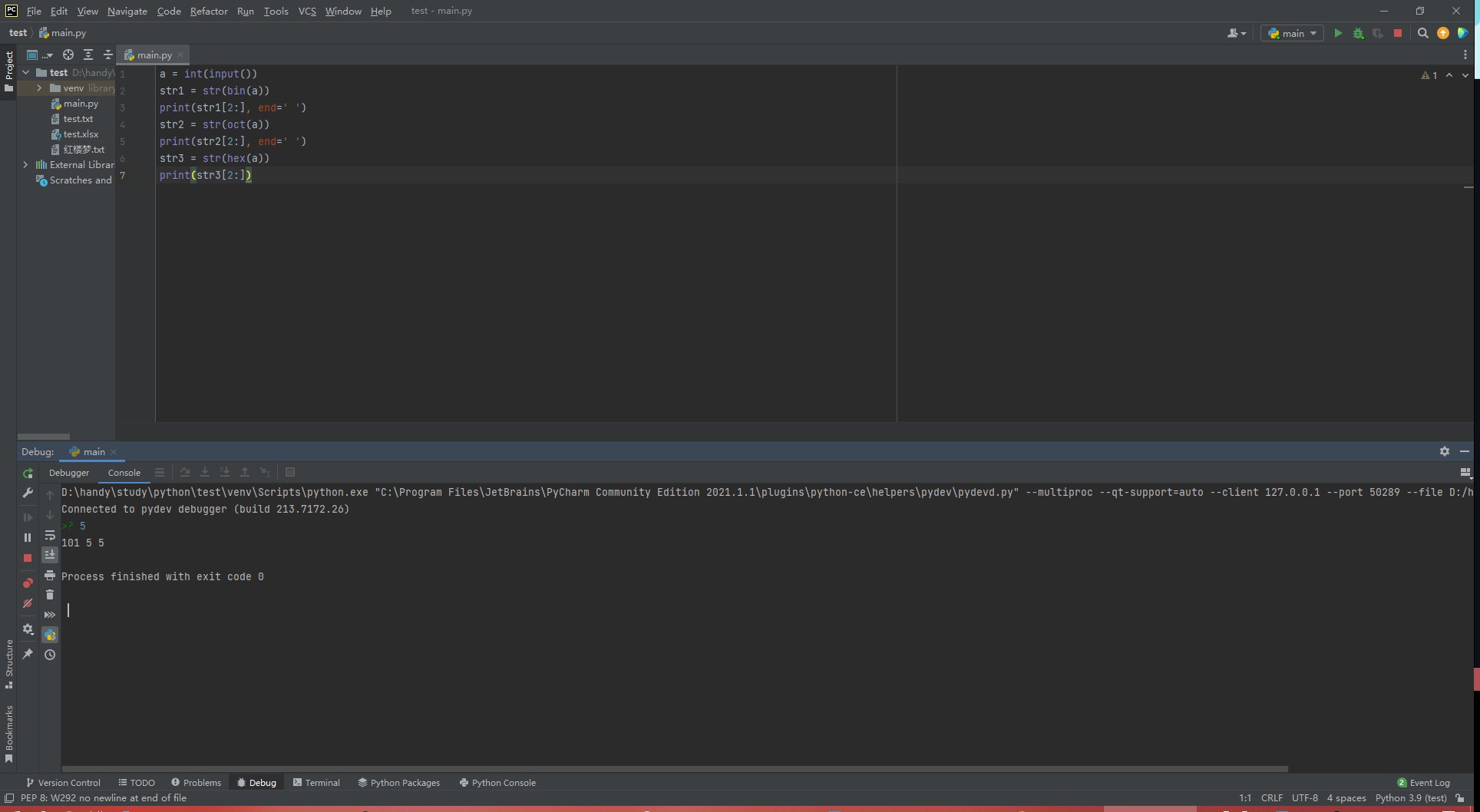###### Try to find the MAX/MIN/AVERAGE num from a num list!

`n = int(input()) list1 = list(map(eval,input().split())) Max = str(max(list1)) print('max=%s' % Max) Min = str(min(list1)) print('min=%s' % Min) sum = 0 for i in range(n): sum = sum + list1[i] Avg = sum/n print('average=%.2f' % Avg)`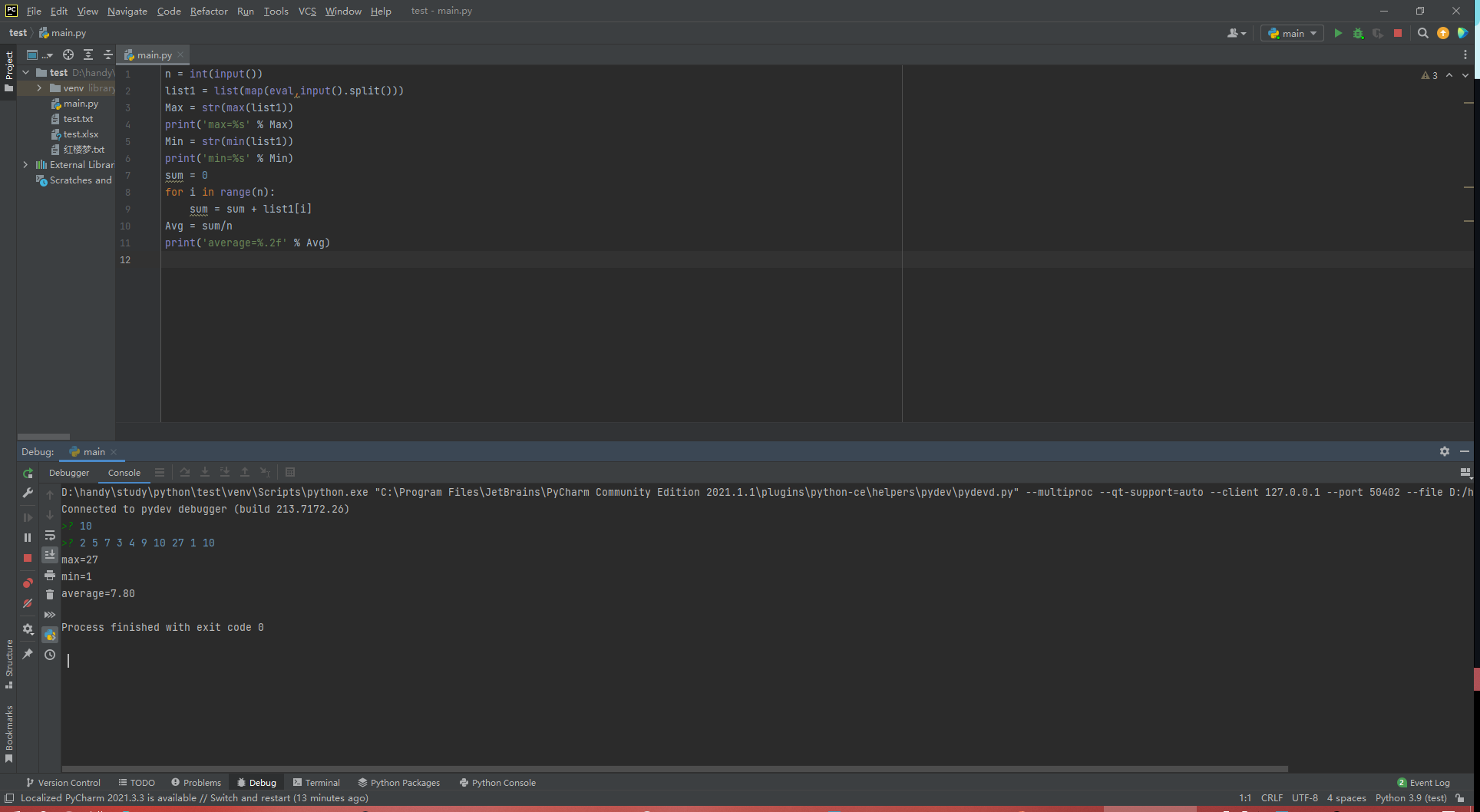##### And this is my first lesson to study Python, I got really much, see u next time!